# 机器学习系列(15)_SVM碎碎念part3：如何找到最优分离超平面

• 如何找到最优超平面
• 如何计算两超平面间的距离
• SVM的最优化问题是什么

## 2.如何找到最优超平面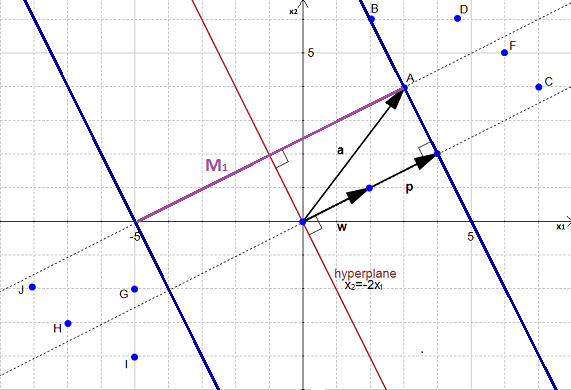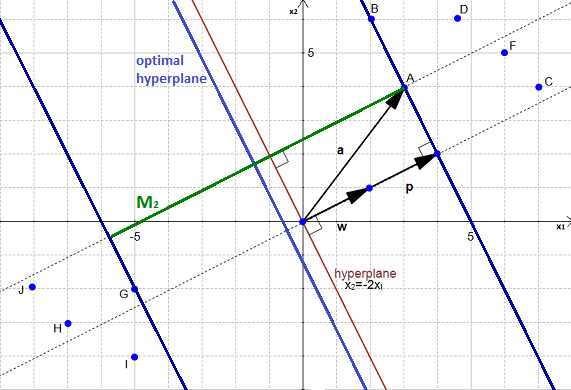• 寻找最大间隔，就是寻找最优超平面

## 3.我们如何找到最大间隔

• 读取你的数据集。
• 找到两个平行超平面，可以划分数据并且两平面之间没有数据点。
• 最大化上述两个超平面间隔

OK，按照上面的步骤，咱们一步步看吧。

### 步骤1：读取想分类的数据集$\mathcal{D}$

={(xi,yi)xip,yi{1,1}}ni=1

### 步骤2：找到两个平行超平面，可以划分数据并且两平面之间没有数据点。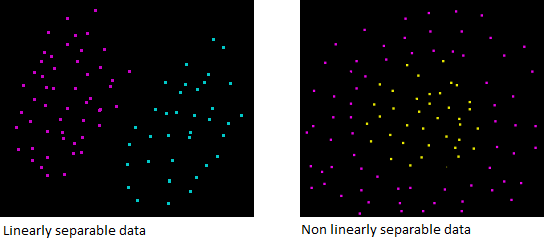wTx=0

wx=b×(1)+(a)×x+1×ywx=yaxb(1)

wx=(a)×x+1×ywx=yax(2)

wxb=yaxbwxb=wx(3)

wxb=0

wxb=δ
wxb=δ

wxb=1wxb=1

wxib1for xi1(4)

wxib1for xi1(5)

xi=A$\mathbf{x_i} = A$我们看到超平面上的点满足wxib=1$\mathbf{w}\cdot\mathbf{x_i} - b =1$，同样的B点也是。

xi=C$\mathbf{x_i} = C$我们看到超平面上的点满足wxib>1$\mathbf{w}\cdot\mathbf{x_i} - b >1$，同样的D、E、F、G点也是。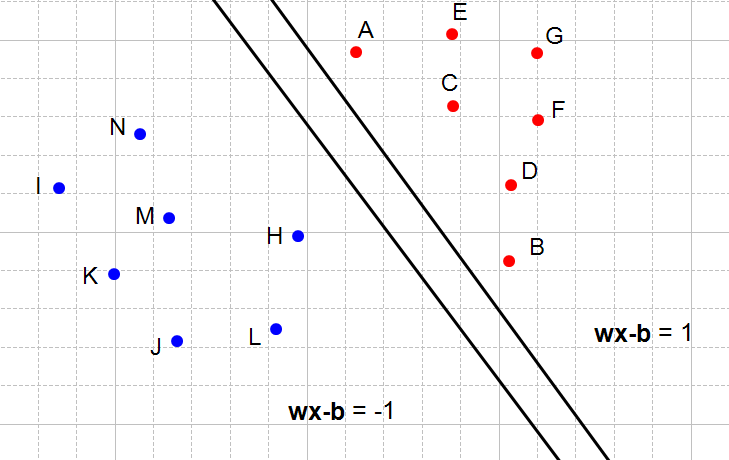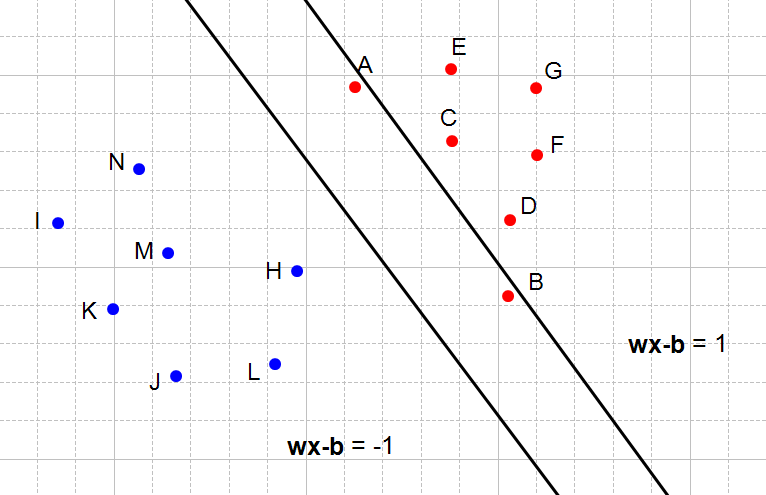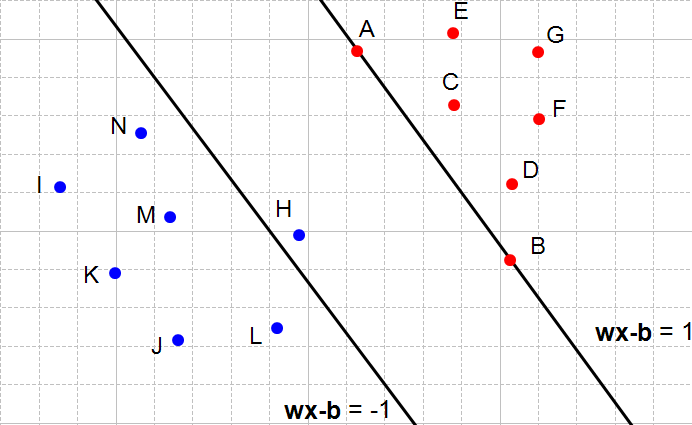OK，所以到此为止，我们找到了一个约束条件–从而能够选择两个中间没有数据点的超平面。当然，这里举得例子是二维平面的，而实际上对于更高维的空间也是成立的。

for xi1wxib1

yi(wxib)yi(1)

yi(wxib)1for xi1(6)

yi(wxib)1for xi1(7)

yi(wxib)1for all1in(8)

OK，我们得到了一个很简洁的约束条件（方程8），它和方程4，5在数学上是等价的，也能保证两超平面间没有数据点。

### 步骤3：两个超平面之间的距离最大化

a)我们的两个超平面之间的距离是什么

OK，现在要做的事情是最大化两个超平面之间的距离，那两个超平面之间的距离怎么计算呢？好，一步步来，我们先做一些标定：

• 0$\mathcal{H}_0$是满足约束wxb=1$\textbf{w}\cdot\textbf{x} - b = -1$的超平面
• 1$\mathcal{H}_1$是满足约束wxb=1$\textbf{w}\cdot\textbf{x} - b = 1$的超平面
• x0$x_0$0$\mathcal{H}_0$上的一点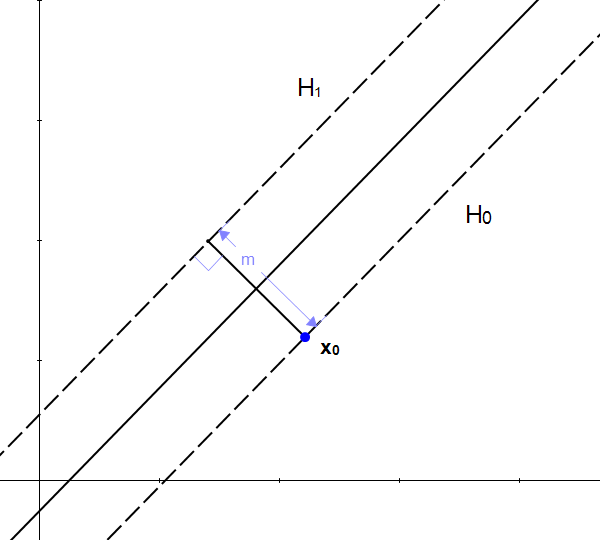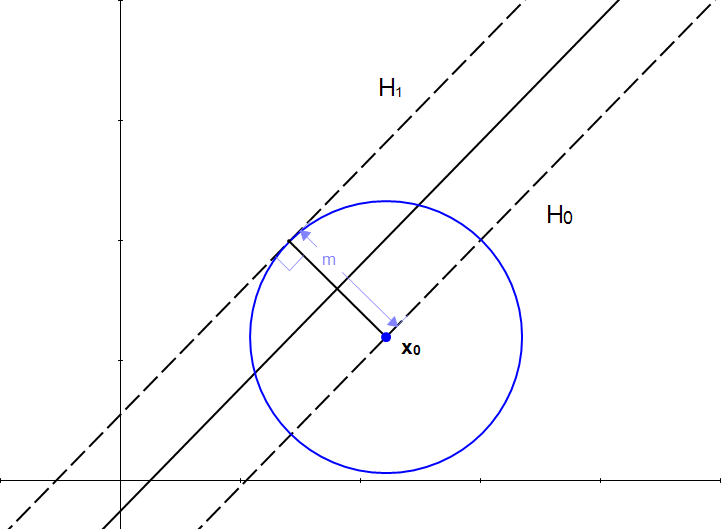OK，结合这张图，你就更清楚了，我们有长度m而缺少另外一个向量的关键信息：方向

OK，标量加向量是没法算了，但大家都知道，向量乘以标量，会得到另外一个缩放后的向量。

OK，所以我们其实是想要找到这样一个向量：

• 长度为m
• 方向垂直于平面1$\mathcal{H}_1$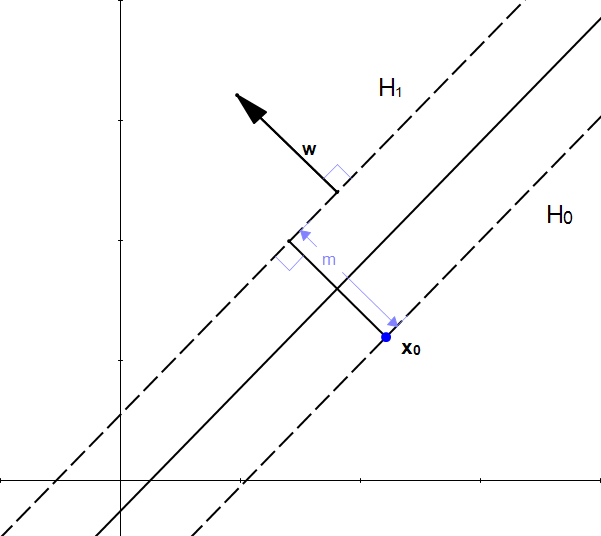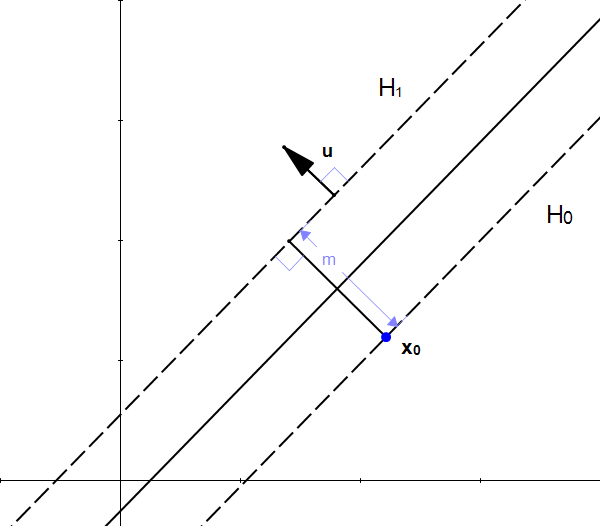• k=m$\|\textbf{k}\| = m$
• k$\textbf{k}$垂直于1$\mathcal{H}_1$，因为它与u$\textbf{u}$具有相同方向

k$\textbf{k}$就是我们正在寻找的向量。k=mu=mww(9)z0$\textbf{z}_0$1$\mathcal{H}_1$上意味着

wz0+b=1(10)

w(x0+k)+b=1(11)

w(x0+mww)+b=1(12)

wx0+mwww+b=1(13)

wx0+mw2w+b=1wx0+mw+b=1wx0+b=1mw

1=1mwmw=2m=2w

OK，赞，我们找到了一种计算m$m$的方法

b) 如何最大化两个超平面之间的距离

m=2w

w=1$\|\textbf{w}\|=1$时，m=2$m=2$
w=2$\|\textbf{w}\|=2$时，m=1$m=1$
w=4$\|\textbf{w}\|=4$时，m=12$m=\frac{1}{2}$

yi(wxib)1i=1,,nww,b

## 4.结论

©️2019 CSDN 皮肤主题: 编程工作室 设计师: CSDN官方博客